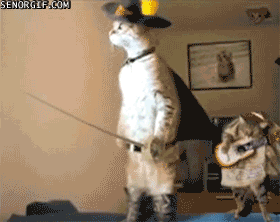# April 20th

### The ideas

• $$n^r$$ is the number of ways of putting $$r$$ distinct marbles into $$n$$ numbered boxes (as many as you would like per box). (Think: each marble has $$n$$ choices.)
• $$n! = n \cdot (n-1) \cdots 2 \cdot 1$$ is the number of ways of putting $$n$$ distinct marbles in $$n$$ numbered boxes (one per box). (Think: after the first marble has a box, the next marble has one less option.)
• $$P(n,r) = \frac{n!}{(n-r)!} = n \cdot (n-1) \cdots (n-r+1)$$ is the number of ways of selecting $$r$$ items from $$n$$ distinct options when order matters. As a marble problem: $$r$$ distinct marbles into $$n$$ boxes, one per box. (Think: first marble has $$n$$ choices, next $$n-1$$, etc.)
• $$\binom{n}{r} = \frac{n!}{(n-r)! r!}$$ is the number of ways of selecting $$r$$ items from $$n$$ distinct options when order does not matter. (The above divided by $$r!$$.) As a marble problem: $$r$$ identical marbles into $$n$$ boxes one per box. (Think: just select $$r$$ boxes out of $$n$$.)
• $$\binom{n+r-1}{n-1}$$ is the number of ways to put $$r$$ identical marbles into $$n$$ boxes. (Think of a string with $$r$$ a's and $$n-1$$ b's, it makes/is/maps to an allocation. e.g. aabaaabaaaa would put 2 marbles in box 1, 3 in box 2, 4 in box 3.)
• With many types of identical marbles: $$r_1$$ of type 1, $$r_2$$ of type 2, $$\ldots$$, $$r_k$$ of type k into $$n$$ boxes is $$\frac{n!}{r_1! \cdots r_k!}$$. This is the number of ways of rearranging the letters in a word (with repeated characters). (Think: normal permutations but divide by all of the double counting that happens when things you thought were different were the same.)

### The problems

1. Four cats and five mice enter a race. In how many ways can they finish with a mouse placing first, second, and third?
2. How many permutations of the letters a,b,c,d,e,f,g contain neither the string bge nor the string eaf?
3. How many numbers with seven distinct digits can be formed using only the digits 2-9?
4. How many different signals, each consisting of seven flags arranged in a column, can be formed from three identical red flags and four identical blue flags?
5. A group of eight scientists is composed of five mathematicians and three geologists.
• In how many ways can five people be chosen to visit a party? (You know, to conduct a study.)
• Suppose the five people chosen to visit the party must be comprised of three mathematicians and two geologists. (It's an upper end affair.) Now in how many ways can the group be chosen?
6. In how many ways can a team of six be chosen from 20 players so as to:
• Include both the strongest and weakest player?
• Include the strongest and exclude the weakest?
• Exclude both the strongest and weakest player?
7. Let $$k$$ and $$n$$ be natural numbers with $$k \lt n$$. Prove, using logic not formulas, that $$\binom{n}{k} = \binom{n-1}{k-1} + \binom{n-1}{k}$$
8. In how many ways can 30 identical dolls be placed on seven different shelves?
9. A florist sells roses in five different colors.
• How many bunches of a half-dozen roses can be formed?
• How many bunches of a half-dozen can be formed if each bunch must contain at least one rose of each color?
10. In how many ways can 18 different books be given to Tara, Danny, Shannon, and Mike so that one person has 6 books, one has 2 books, the other two people have 5 books each?

### A cat gif